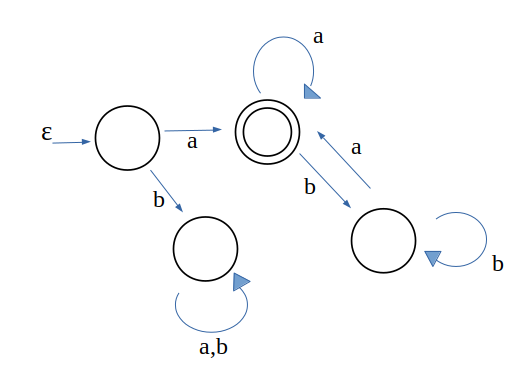# Program to build DFA that starts and end with ‘a’ from input (a, b)

DFA (Deterministic Finite Automaton or Acceptor) is a finite state machine that accepts or rejects strings of symbols. DFA accepts the string if it reaches the final state and rejects otherwise.

Now the problem is, provided a string as input character by character and we have to check whether the string starts and ends with ‘a’. We can only store the current character, since there is no concept of memory and hence the DFA cannot store the string provided. Otherwise, we could have just checked the first and last character for this problem. The input set for this problem is (a, b).

We cannot store anything accept the current character, which make this program a little different and tough than other string related problems.

Examples:

```Input :  a b a b a
Output : Yes
Explanation : (a b a b a) starts and
end with 'a'

Input :  a b a b b
Output : No
Explanation : (a b a b b) starts with
'a' but doesn't end with 'a'
```

## Recommended: Please try your approach on {IDE} first, before moving on to the solution.

We first build a DFA for this problem. Making DFA is like making a flowchart for this program and then implement it in any language. You should have the knowledge of DFA and Finite Automata.

The DFA for given problem is:`// CPP Program to DFA that accept strings ` `// which starts and end with 'a' over input(a, b) ` `#include ` `#include ` `using` `namespace` `std; ` ` `  `int` `main() ` `{ ` `    ``// for producing different random  ` `    ``// numbers every time. ` `    ``srand``(``time``(0)); ` ` `  `    ``// random length of string from 1 - 16 ` `    ``// we are taking input from input stream, ` `    ``// we can take delimiter to end the string ` `    ``int` `max = 1 + ``rand``() % 15; ` ` `  `    ``// generating random string and processing it ` `    ``int` `i = 0; ` `    ``while` `(i < max) { ` ` `  `        ``// producing random character over ` `        ``// input alphabet (a, b) ` `        ``char` `c = ``'a'` `+ ``rand``() % 2; ` `        ``cout << c << ``" "``; ` `        ``i++; ` ` `  `        ``// first character is 'a' ` `        ``if` `(c == ``'a'``) { ` ` `  `            ``// if there is only 1 character ` `            ``// i.e. 'a' ` `            ``if` `(i == max) ` `                ``cout << ``"YES\n"``; ` ` `  `            ``while` `(i < max) { ` `                ``c = ``'a'` `+ ``rand``() % 2; ` `                ``cout << c << ``" "``; ` `                ``i++; ` ` `  `                ``// if character is 'a' and it ` `                ``// is the last character ` `                ``if` `(c == ``'a'` `&& i == max) { ` `                    ``cout << ``"\nYES\n"``; ` `                ``} ` ` `  `                ``// if character is 'b' and it  ` `                ``// is the last character ` `                ``else` `if` `(i == max) { ` `                    ``cout << ``"\nNO\n"``; ` `                ``} ` `            ``} ` `        ``} ` ` `  `        ``// first character is 'b' so no matter ` `        ``// what the string is, it is not going  ` `        ``// to be accepted ` `        ``else` `{ ` `            ``while` `(i < max) { ` `                ``c = ``'a'` `+ ``rand``() % 2; ` `                ``cout << c << ``" "``; ` `                ``i++; ` `            ``} ` `            ``cout << ``"\nNO\n"``; ` `        ``} ` `    ``} ` ` `  `    ``return` `0; ` `} `

Output:

```a a b a a
YES
```

My Personal Notes arrow_drop_upCheck out this Author's contributed articles.

If you like GeeksforGeeks and would like to contribute, you can also write an article using contribute.geeksforgeeks.org or mail your article to contribute@geeksforgeeks.org. See your article appearing on the GeeksforGeeks main page and help other Geeks.

Please Improve this article if you find anything incorrect by clicking on the "Improve Article" button below.

Article Tags :
Practice Tags :

1

Please write to us at contribute@geeksforgeeks.org to report any issue with the above content.